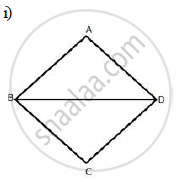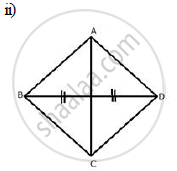# The Locus of a Point in Rhombus Abcd, So that It is Equidistant from (I) Ab and Bc; (Ii) B and D. - Mathematics

Sum

Describe the locus for questions 1 to 13 given below:

The locus of a point in rhombus ABCD, so that it is equidistant from
(i) AB and BC; (ii) B  and D .

#### SolutionThe locus of the point in a rhombus ABCD which is equidistant from AB and BC will be the diagonal BD.The locus of the point in a rhombus ABCD which is equidistant from B and D will be the diagonal AC.

Concept: Theorems Based on Loci
Is there an error in this question or solution?

#### APPEARS IN

Selina Concise Maths Class 10 ICSE
Chapter 16 Loci (Locus and Its Constructions)
Exercise 16 (B) | Q 13 | Page 241